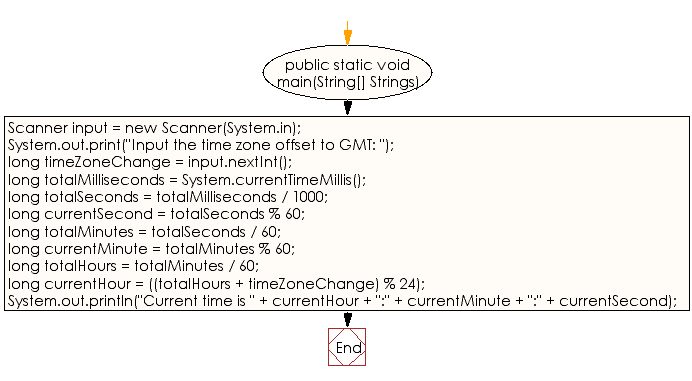﻿ Java exercises: Print the current time in GMT - w3resource# Java Data Type Exercises: Print the current time in GMT

## Java Data Type: Exercise-5 with Solution

Write a Java program that prints the current time in GMT.

GMT: Greenwich Mean Time (GMT) is the mean solar time at the Royal Observatory in Greenwich, London. GMT was formerly used as the international civil time standard, now superseded in that function by Coordinated Universal Time (UTC).

Test Data
Input the time zone offset to GMT: 256

Sample Solution:

Java Code:

``````import java.util.Scanner;
public class Exercise5 {

public static void main(String[] Strings) {

Scanner input = new Scanner(System.in);

System.out.print("Input the time zone offset to GMT: ");
long timeZoneChange = input.nextInt();

long totalMilliseconds = System.currentTimeMillis();

long totalSeconds = totalMilliseconds / 1000;

long currentSecond = totalSeconds % 60;

long totalMinutes = totalSeconds / 60;

long currentMinute = totalMinutes % 60;

long totalHours = totalMinutes / 60;

long currentHour = ((totalHours + timeZoneChange) % 24);

System.out.println("Current time is " + currentHour + ":" + currentMinute + ":" + currentSecond);
}
}
```
```

Sample Output:

```Input the time zone offset to GMT: 256
Current time is 5:7:51
```

Flowchart:Java Code Editor :

Improve this sample solution and post your code through Disqus

What is the difficulty level of this exercise?

﻿

## Java: Tips of the Day

Checks if a string is upper case:

```public static boolean isUpperCase(String input) {
return Objects.equals(input, input.toUpperCase());
}
```

Ref: https://bit.ly/39Hpo84